# Perimeter of Circle

In Perimeter of circle lesson, we are going to discuss,

• What is the Perimeter of circle or circumference of circle

• Circumference equation or circumference formula

• Perimeter of semicircle
• Perimeter of quarter circle
• Perimeter of sector

## What is the Perimeter of Circle

Before moving into perimeter let’s recall some knowledge about key features of circle.

• Center of Circle
• Diameter of Circle

### Center of Circle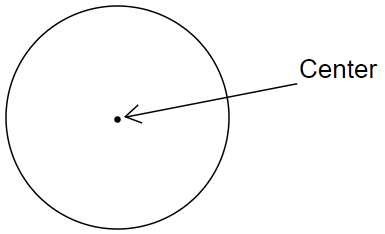The center is the exact midpoint of the circle.

In other words, we can also define the Center as the point at which the axis of symmetry of a circle intersects is the center.### Diameter of Circle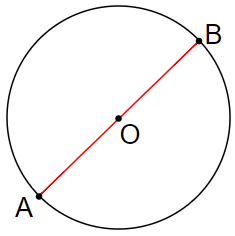Diameter is a straight segment joining two points on a circle, which passes through the center of the circle.

In this circle, A B is the diameter.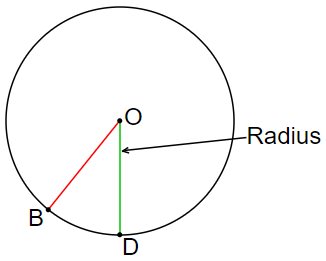The straight line segment joining the center to any point on the circle is called a Radius of a circle.

If the center is ‘O’ and the point on the circle is ‘B’ then, OB is the radius of the circle.

OB and OD are ‘Radii’ (plural form of the radius.) of the circle.

There is a relationship between diameter and radiusDiameter AB

AB = AO + BO

AB = AO + AO ; because BO = AO

AB = 2 AO

The diameter of a circle is twice it’s  radius.

#### The simple meaning of perimeter is length around a closed plane figure

When it comes to circles we also called it the circumference of circle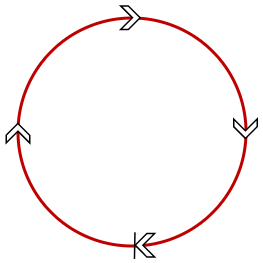## Circumference Formula

### For full circle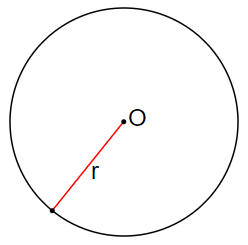If ‘O’ is the center and ‘r’ is the radius of this circle.

The circumference ‘c’ will be,

c = 2πr

Here π is a constant value.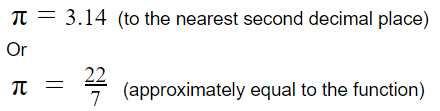Example 01:

Find the circumference of a circle of radius 14cm.

Circumference  c = 2πr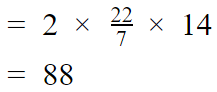Therefore the circumference is 88cm.

When the diameter is given instead of the radius we have another circumference equation.

Let ‘d’ be the diameter and ‘r’ is the radius.

d = 2πr (two times radius equal to diameter)

If the circumference of the circle denoted by ‘c

c = 2πr

We can also write circumference of a circle formula as,

c = 2πr

c = π x d    (d = 2πr)

c = πd

Example 02:

Find the circumference of a circle of diameter 21cm.

#### This example is about the diameter to circumference.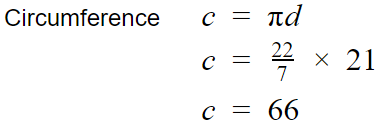Therefore the circumference is 66cm.

### Exercise 01

Find the circumference of the circle with the measurements given below.

2.  Diameter 28m (hint: convert into mixed number)
3.  Diameter 1712cm

### Perimeter of Semicircle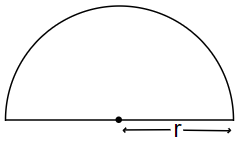When a circular lamina separated into two equal parts along a diameter each part is known as a semicircle.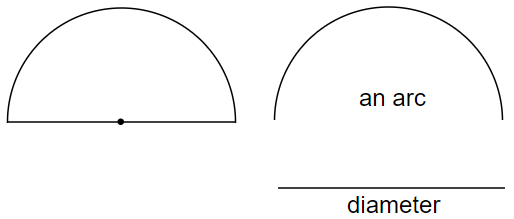The arc length of the semicircle of radius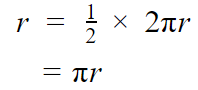It is clear from the figure that, to find the perimeter of a semicircle, the diameter should be added to the arc length.

The perimeter of a semicircle of radius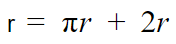Example 03:

Find the perimeter of semicircle when the diameter is 21cm.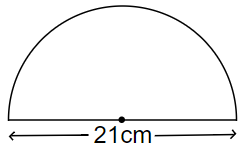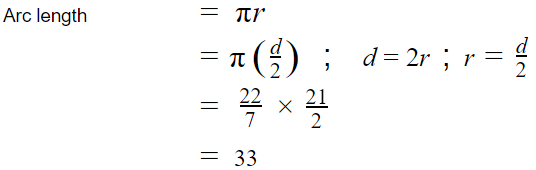You can refer multiplication of fractions article to learn simplifications.

2r = diameter = 21cm

Therefore the perimeter of the semicircle

= 33cm + 21cm

= 54cm

### Exercise 02:

Find the perimeter of the semi-circular lamina with the measurements given below.

2. Diameter = 7cm

### Perimeter of Quarter Circle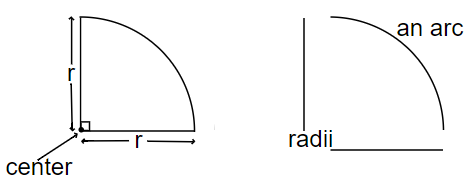The arc length of the quarter circle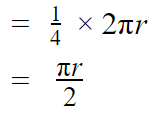It is clear from the figure that, to find the perimeter of a quarter circle, two radii should add to the arc length.

The perimeter of quarter circle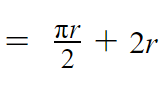Example 04:

If the radius is 28cm, find the perimeter of the quarter circle.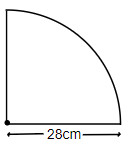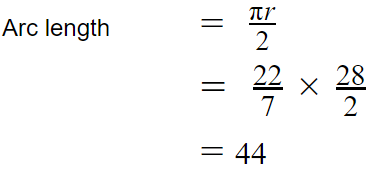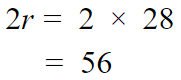Therefore the perimeter of the quarter circle

= 44cm + 56cm

= 100cm

### Perimeter of a Circle Sector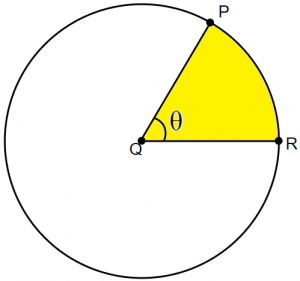A region bounded by two radii and an arc is called a sector of a circle.

The angle subtended at the center of the circle by the arc is called the central angle.

Here the central angle is θ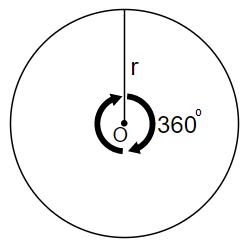The angle at the center of the circle is 360o. The arc length related to the 360o angle is r.

By looking at the above diagram we can say that,

For 360o the arc length will be r.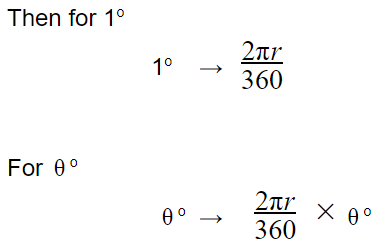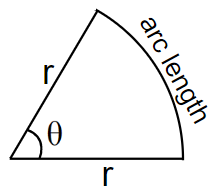The arc length of a sector of a circle with radius ‘r’ and central angle ‘π.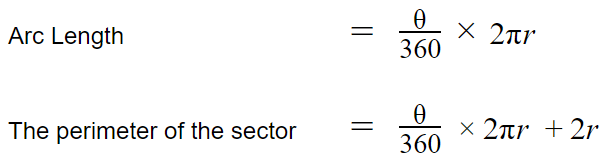Example 05:

Find the perimeter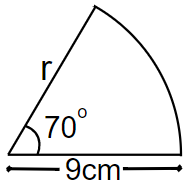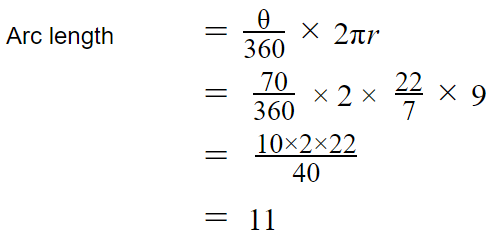Arc length is 11cm.

Therefore the perimeter of the sector

= 11cm + 2 x 9cm

= 11cm + 18cm

= 29cm

#### Exercise 01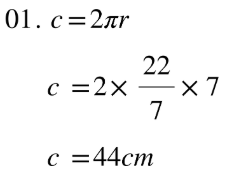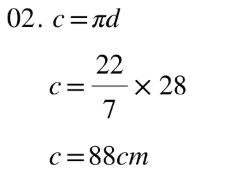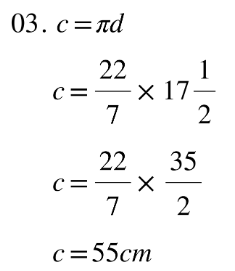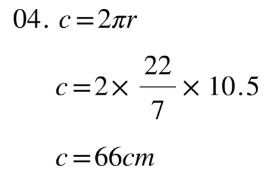#### Exercise 02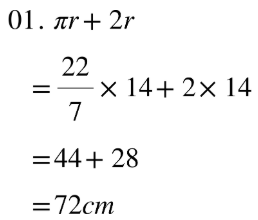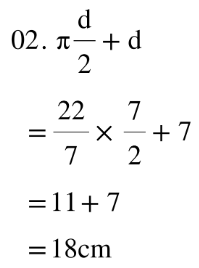## Calculator for Semi Circles - Area and Perimeter

Using this calculator, you can find the area and perimeter of semi-circles.

Radius, diameter, Perimeter and area calculation option are available. Select what you need to find and enter the number in text box. Then click ‘submit’.

Always remember this calculator is only checking for your answers. You have to do the exercises your self.